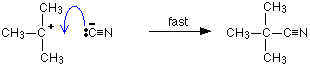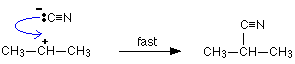# E. Substitution Reactions Involving Cyanide Ions

$$\newcommand{\vecs}{\overset { \rightharpoonup} {\mathbf{#1}} }$$ $$\newcommand{\vecd}{\overset{-\!-\!\rightharpoonup}{\vphantom{a}\smash {#1}}}$$$$\newcommand{\id}{\mathrm{id}}$$ $$\newcommand{\Span}{\mathrm{span}}$$ $$\newcommand{\kernel}{\mathrm{null}\,}$$ $$\newcommand{\range}{\mathrm{range}\,}$$ $$\newcommand{\RealPart}{\mathrm{Re}}$$ $$\newcommand{\ImaginaryPart}{\mathrm{Im}}$$ $$\newcommand{\Argument}{\mathrm{Arg}}$$ $$\newcommand{\norm}{\| #1 \|}$$ $$\newcommand{\inner}{\langle #1, #2 \rangle}$$ $$\newcommand{\Span}{\mathrm{span}}$$ $$\newcommand{\id}{\mathrm{id}}$$ $$\newcommand{\Span}{\mathrm{span}}$$ $$\newcommand{\kernel}{\mathrm{null}\,}$$ $$\newcommand{\range}{\mathrm{range}\,}$$ $$\newcommand{\RealPart}{\mathrm{Re}}$$ $$\newcommand{\ImaginaryPart}{\mathrm{Im}}$$ $$\newcommand{\Argument}{\mathrm{Arg}}$$ $$\newcommand{\norm}{\| #1 \|}$$ $$\newcommand{\inner}{\langle #1, #2 \rangle}$$ $$\newcommand{\Span}{\mathrm{span}}$$$$\newcommand{\AA}{\unicode[.8,0]{x212B}}$$

## Reacting Primary Halogenoalkanes with Cyanide Ions

If a halogenoalkane is heated under reflux with a solution of sodium or potassium cyanide in ethanol, the halogen is replaced by a -CN group and a nitrile is produced. Heating under reflux means heating with a condenser placed vertically in the flask to prevent loss of volatile substances from the mixture. The solvent is important. If water is present you tend to get substitution by -OH instead of -CN.

For example, using 1-bromopropane as a typical primary halogenoalkane:

$CH_3CH_2CH_2Br + CN^- \rightarrow CH_3CH_2CH_2CN + Br^-$

The full equation could be written rather than the ionic one, but it obscures what's going on:

$CH_3CH_2CH_2Br + KCN \rightarrow CH_3CH_2CH_2CN + KBr$

The bromine (or other halogen) in the halogenoalkane is simply replaced by a -CN group - hence a substitution reaction. In this example, butanenitrile is formed. Here is the mechanism for the reaction involving bromoethane:This is an example ofnucleophilic substitution. Because the mechanism involves collision between two species in the slow step (in this case, the only step) of the reaction, it is known as an SN2 reaction. If your examiners want you to show the transition state, draw the mechanism like this:## Reacting Tertiary Halogenoalkanes with Cyanide Ions

The facts of the reaction are exactly the same as with primary halogenoalkanes. If the halogenoalkane is heated under reflux with a solution of sodium or potassium cyanide in ethanol, the halogen is replaced by -CN, and a nitrile is produced.

For example:

$(CH_3)_3CBr + CN^- \rightarrow (CH_3)_3CCN + Br^-$

Or if you want the full equation rather than the ionic one:

$(CH_3)_3CBr + KCN \rightarrow (CH_3)_3CCN + KBr$

This mechanism involves an initial ionization of the halogenoalkane:followed by a very rapid attack by the cyanide ion on the carbocation (carbonium ion) formed:This is again an example of nucleophilic substitution. This time the slow step of the reaction only involves one species - the halogenoalkane. It is known as an SN1 reaction.

## The reaction of secondary halogenoalkanes with cyanide ions

The facts of the reaction are exactly the same as with primary or tertiary halogenoalkanes. The halogenoalkane is heated under reflux with a solution of sodium or potassium cyanide in ethanol.

For example:Secondary halogenoalkanes use both SN2 and SN1 mechanisms. For example, the SN2 mechanism is:The two stages of the SN1 mechanism are:## Contributors

This page titled E. Substitution Reactions Involving Cyanide Ions is shared under a CC BY-NC 4.0 license and was authored, remixed, and/or curated by Jim Clark.